﻿ Hzao's Blog – 第4页 – Based on WordPress

## 数列分块心得

//作者过弱， 此文过水， 不建议阅读

### 关于标记的用法

1.在查询整体和的时候使用。（李煜东的书给出的就是这种）

2.查询部分元素的时候使用。

## Hash

Hash表也叫散列表，可以将复杂而庞大的数据映射到一定的小范围内。缺点是可能出现冲突，但可以用链表的方法解决。

10003不是质数。它等于7*1429；10007是，但100007不是，它等于97*1031；

#### 字符串Hash

inline bool cmp(int a,int b){     int mid,l=0,r=min(len-a+1,len-b+1);     while(l>1;         if(eql(a,a+mid-1,b,b+mid-1)){//记得-1 求的是长度             l=mid;         }         else r=mid-1;     }     return s[a+l]<s[b+l]; }

inline bool eql(int l1,int r1,int l2,int r2){      return f[r1]-f[l1-1]p[r1-l1+1]==f[r2]-f[l2-1]p[r2-l2+1];

## 出栈的合法顺序

（这是一篇水贴）

         int A=1,B=1;
stack s;
while(A<=n){
if(a[A]==B){
A++;B++;
}
else if(!s.empty()&&s.top()==a[A]){
s.pop();
A++;
}
else if(B<=n){
s.push(B++);
}
else {
break;//不合法
}
}

      inline void dfs(int s) {
if(cnt == n) {
num++;
/*
for (int i = 1;i <= n; ++i) printf("%d", c[i]);
printf("\n");
*/
return;
}
if (top > 0) {
int tp = a[top--];
c[++cnt] = tp;
dfs(s);
a[++top] = tp;
cnt--;
}
if (s <= n) {
a[++top] = s;
dfs(s+1);
top--;
}
}
int main(){
scanf("%d", &n);
dfs(1);
printf("%d", num);
return 0;
}


## 矩阵乘法

#### 运算方法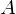矩阵和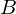矩阵乘积中的元素,等于矩阵第行的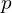个数和矩阵第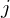列的个数分别相乘的和。 即：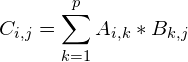#### 纯乘法的代码实现

1.计算函数。由于自己太弱了，加上时间有限，写了一份很水的代码。

#include<cstdio>
int A={
{1,2,3},
{4,5,6}
};
int B={
{1,2,3,4},
{5,6,7,8},
{9,10,11,12}
};
int c;
int n,m;
void mul(int a[],int b[],int n,int m,int p){
for(int i=0;i<n;++i){//n 乘积的行数
for(int j=0;j<m;++j){//m 乘积的列数
for(int k=0;k<p;++k){//p 即共有的维
c[i][j]+=a[i][k]*b[k][j];
}
printf("c[%d][%d]=%d\n",i,j,c[i][j]);
}
}
}
int main(){
mul(A,B,2,4,3);//计算A*B,并保存到c[]数组中
return 0;
}

2.有一种边读入边计算的写法：

	scanf("%lld%lld%lld",&n,&p,&m);
for(long long i=1;i<=n;++i){
for(long long j=1;j<=p;++j){
scanf("%lld",&a[i][j]);
}
}
for(long long i=1;i<=p;++i){
for(long long j=1;j<=m;++j){
scanf("%lld",&b[i][j]);
for(long long k=1;k<=n;++k){//此处k枚举的并非是公共维
ans[k][j]+=a[k][i]*b[i][j];
}
}
}

#### 用途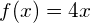之类的线性函数的推广。

——维基百科

1.其实蒟蒻的我并不知道怎么解线性方程组，但是对于矩阵加速线性变换有一些体会。让我们考虑一种比较特殊的矩阵乘法：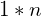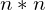。由定义可知，它们的乘积仍然是一个的矩阵。那么有什么用呢？我们可以借助快速幂的思想，利用矩阵乘法的分配律、结合律，降低时间复杂度。2.以后遇到再来补充。